## Otolaryngology Online Journal

All submissions of the EM system will be redirected to Online Manuscript Submission System. Authors are requested to submit articles directly to Online Manuscript Submission System of respective journal.
Reach Us+44 151 808 1136

Review Article - Otolaryngology Online Journal (2017) Volume 7, Issue 2

# Improved Ant Colony Algorithms for Multi-agent Path Planning in Web3D Environment

*Corresponding Author:
Fengting Yan, DMedSc, Xi’an Medical University, China, Tel: +86 29 8266 8888, E-mail: yanfengting2008@163.com

Received date: March 07, 2017; Accepted date: April 12, 2017; Published date: April 20, 2017

Visit for more related articles at Otolaryngology Online Journal

## Abstract

The path planning in 3D large-scale scenes is a hard problem. Especially, there are many multi-agents searching their optimal paths in a complex and large-scale 3D scene. This paper improves two kinds of ant colony algorithms and a leader idea to solve the problem. Firstly, an improved 2D ant colony algorithm for flat path planning is proposed. It is a method based on direction to choice a point as the next step point to make path planning precision. Secondly, a classic 3D ant colony algorithm is improved for mountain terrain path planning. We put forward a concept of vertebral visual area, which accelerates the speed of path finding in looking for the next node locked area. At last, a leader idea was given for multi-agent path planning when soldiers are marching in a mountain. And in every improved method, an experiment was done which displayed that the convergence is fast, and the path planning results are efficient, real time and precise.

## Keywords

Multi-agent path planning, hybrid ant colony algorithm, large-scale Web3D

## Introduction

Path planning includes global path planning  and local path planning . Global path planning refers to making optimal path planning based on the environment data. Local planning refers to the path planning without the environment data or where only part of the environment data is available. Local path planning has some limitations in some places for the position data of all the obstacles in the unknown environment, because the algorithm process is computationally expensive. For example, in mountain terrain there are many soldiers marching, the path planning need more calculation than global path planning. As we know, the terrain of our earth can be divided two kinds. The first kind is flat terrain; the second is mountain terrain.

The question of 3D path planning in a flat terrain can be translated into a 2D ant colony optimal algorithm . In this way, we can improve 2D ant colony optimal algorithm for our 3D flat terrain path planning. The detail method is as follows:

Step 1: Constructing the MAKLINKE graph according to the physical layout of construction equipment in the scene

Step 2: The algorithm uses the Dijkstra algorithm to search for a sub-optimal path.

Step 3: The algorithm uses the improved ant colony algorithm to plan the shortest path.

In order to make path planning to find a satisfied optimal certain indicators from the starting point to the target point in the large-scale 3D scenes, and avoid the obstacle, we use a group of 3D map dates. All the existing path planning methods are mostly in the two-dimensional plane or quasi 2D planning of planar path planning. Due to the complexity of 3D path planning process in calculation, the information storage capacity is huge, and it is difficult to directly make optimal path for global planning problems, so there are more challenging in the process. The existing 3D path planning mainly includes the A* algorithm, genetic algorithm and particle swarm optimization (PSO) algorithm . However the A* algorithm calculation will increase sharply with an increase in dimension number. The Genetic algorithm and particle swarm algorithm is quasi three-dimensional planning algorithm. The ant colony algorithm has the advantage of distributed computing and swarm intelligence, which has great potential in path planning. The ant colony algorithm path planning is in successfully applied in 2D at the same time, which can also be used for 3D path planning.

## Overview

The agent path planning problem is planning a best path from initial state to goal state in accordance with the principle of a certain or potential optimization (such as the shortest path, minimum energy consumption, or the fastest time) in the work environment with obstacles without collision with obstacles .

The intelligent path planning consists of two parts: the environment building and the planning method. In the environment building model, this path planning can be summarized as global path planning and local path planning. Global path planning includes the configuration space method, the generalized cone method, the vertex image method, and the grid tumble method. The local path planning algorithm is mainly the artificial potential field method, etc. There are many kind of agent path planning methods based on the space model, such as: the A* algorithm, the ant colony algorithm, the particle swarm optimization (PSO) algorithm, the genetic algorithm and the neural network algorithm4. There are two aspects that can be improved in the Ant colony algorithm: the first one is the different transfer rules to balance the algorithm performance of exploration and development; Second, the different pheromone update rules. The advantage of the ant colony algorithm is to be able to find good approximate solutions quickly, and the drawback is it can easily lead to premature convergence. We use additional heuristic information to avoid premature convergence.

The intelligent planning algorithm is based on the geometric model of search methods, mainly virtual potential field, the navigation function method, and the mathematical optimization method, etc. All sorts of obstacles are presented in the polygon. If two vertices’ attachment does not intersect with any obstacles, we use an accepting line as a method for solving the candidate path segment, which is called the View method. Kuwata and How has applied this method to UAV path planning. The downside of the View method is that it is usually not the optimal path. The Artificial potential field method is bases on the virtual potential field and navigation function. In the two-dimensional space the method of path planning has been widely used. Its advantage is the simple algorithm, and the execution efficiency is high. Kitamura, etc. referenced the method to 3D path planning. The method using Octree to present the three-dimensional configuration space for agent artificial potential field model is established. Support vector machine (SVM) is a kind of method based on mathematical optimization . The idea of the method is using the interval maximization principle to solve the support vector machine (SVM) classification problem. Got the nonlinear integral plane, and then choose from splitting plane out suitable for smart mobile path .

The path planning methods based on intelligent planning algorithms mainly include: inspired by biological genetics and genetic algorithm, inspired by ant colony foraging behaviour of ant colony algorithm, inspired by birds, fish behaviour of particle swarm optimization (PSO) and inspired by the human brain nervous system of neural network is put forward . Genetic algorithms have a good initial solution under the condition of fast convergence and high quality solutions, but the biggest deficiency of genetic algorithm is their inability to meet realtime requirements . Particle swarm optimization (PSO) algorithm in the known local path planning or unknown three-dimensional path is a quasithree dimensional path planning. Neural network algorithms are good for solving highly nonlinear and uncertainty of system control problems, and this method has great potential, but the algorithm requires a small number of obstacles in the space, because as the number of obstacles in the scene, then the algorithm complexity is greatly increased.

Advanced 2D ant colony path planning algorithm

If the terrain is flat, for example the large-scale underground market space?we would consider the x and y value of agent position because the z value is a constant. So we can use the 2D optimal path planning algorithms .

Firstly, we map the large-scale scene from the real scene into a flat map. This work is to build a spatial model.

Then, we make use of the MAKLINE graph algorithm to establish the 2D space.

Thirdly, in the 2D space, you can give an initial path from the start point to the target point by using the Dijkstra algorithm. Along the initial path, we use the classical ant colony algorithm to optimize the path .

However, there are usually some parts of the path are not the shortest ones. So the paper proposed an improved ant colony algorithm (Figure 1).

Improved 2D Ant Colony Algorithm: To display the improved ant colony algorithm, we abstract a usual scene from our real public world. In this paper, we map the Shanghai south station underground space into a spatial model (Figure 2).

Step 1: Mapping the public scene

In the abstracted public space, there are four irregular shapes of building facilities which divided into A, B, C and D four areas [11,12]. A is dining area, B is business area, C is the entertainment area, including a cinema, and the last area D is for retail and the remainder, entertainment. The unmarked area is free walking, and there are two stair gangways which are M and N. Consider there is a fire disaster near the M gangway, so the agents in the space must be evacuated to the N gangway. We hypothesis the S point is a start for one agent or some agents, so our intelligent ant colony algorithm must find a shortest path for agents to evacuate from the current position. The scope of fire disaster covered, which is set as an obstacle area (Figure 3).

Then we build MAKLINK lines using AS3.0 computer language to construct a path planning space . These lines connect with two obstacles but don’t intersect obstacles . And there are some connecting lines between the vertexes with the environment border (Figure 4).

There is a free curved cable in the MAKLINK figure, and these lines showed with red dotted colour are connected in the middle point in proper order by v1, v2, v20. The start point is S, from which the all middle points are connected by the MAKLINK lines until to the T point. An undirected network diagram initial path planning is constructed. This process is very fast .

Step 3: searching an shortest path using Dijkstra algorithm

Here, we use Dijkstra algorithm to plan a path which can be used to calculate the shortest path of the point in the non-negative weights figure. The base idea is to make use of the power weight to calculate the least all power weight with the initial points.

Computational steps are

Step 1: Dividing all the middle points in the power weight figure into two arrays, the start point is pushed into the V array and the others are put into the S array.

Step 2: Getting all points in the V array together with some points in S array, and we can calculate the total power weight value from the start point S to all the other points. Based on the calculated value to form an array, from which we can choose the least power value point, which will be pushed into the V array as a member point of it.

Step 3: Continue executing step 2 until finding an array of order points which start from S to the target point T, at which point the calculation ends.

The Dijkstra algorithm process keeps calculating the power weight value from V points to the points of S array. By this way, we can calculate the least power weight value from the start point to any other points. Using this algorithm, the path process is as the yellow colour path (The yellow path is the result of Dijkstra Algorithm, and the blue path is the result of ant colony algorithm) [15,16].

The calculation is very fast in this process, and we can get a rough approximation to the shortest path. That can be used in the web for user. To improve the shortest path, we should use ant colony algorithm in the next step.

Step 4: Ant colony algorithm

We can get the Dijkstra path point of S, P1, P2, Pd and T based on the MAKLINE.

Firstly, we need define a unit length of tiny segment of l, every l can be divided into many short lines by, and we can get the number of segment. The division begins from the start point of every line: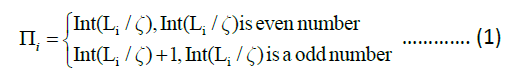Here, is the number of segment by division. Every step in the next point searching process, the ant chooses its path from li to li+1 by Pij, and using the roulette method to select a point. This decides the next step path for the path. To avoid premature convergence, we make use of the method of the initial pheromone values decrease, and after a finished path finding process, the shortest path pheromone value increases. In this method, the ant colony algorithm can avoid the premature convergence for searching the shortest path, and access the velocity of finding optimal path (Figure 5).

The process of point choice is optimized by ant colony algorithm, and we find the optimal path (Figure 6). Here, the yellow path shows the Dijkstra algorithm and the blue path the ant colony algorithm.

Through the ant colony optimization algorithm, the path aided by Dijkstra Algorithm is shortened. However, the path is not close the building in the red area of A. At the same time, it is not the shortest path planning in the B area. So the base ant colony algorithm needs to be improved.

Step 5: Improved Ant Colony Algorithm

In the finding process of the ant colony, when an ant is at the position of pi, then it search for the next point of pi+1, the basic ant colony algorithm is based on the probability calculation of pheromone and distance which start from pi to the next point on the next line. Because every point choice is to decrease the total length from current point to the end point, here we choice the nearest point leaving that point of intersection O. from the Figure 5, we can find the pi+1 is the nearest point which is in the line of pi1pi2. So the best point should be pi+1.

Here, pi1 and pi2 are the two endpoints, and the S is the begin of the path planning, and T is the end of the path planning; the pi is the current point of an ant, and the pi+1 point is the searching point of the current ant. The red point O is the intersection point of the ST line together with the pi1pi2 line.

At the pi+1 point, the update should do for the pheromone: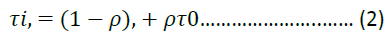The t0 as pheromone initial value, and the p is as adjustable parameter in [0,1] section.

When all ants have travelled through the path from start to the end, and completed the iterative search in turn, we can choose the shortest path from all the paths, and to update the pheromone in the shortest path, that is:of which, the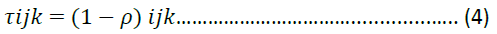? and the L* is the length of the shortest path.

The improved ant colony algorithm is shown on the algorithm procedures in the next figure; here is the main part of our improved algorithm:

 Node initialization Begin For every current point in the line { Calculate the point of ST and pi1pi2; Calculate all distance from the intersection point O; The nodes in order; If (Judging the passable of the minimum distances point); The point pi+1 is the optimal path point; } End

Experiment result

The optimal path by improving ant colony algorithm: In Figure 6, we can find that the path is shorter than the basic ant colony algorithm. The path planed by the improved ant colony algorithm is close to the building. And in the area of B, the path is shorter than the basic ant colony algorithm. So the improved ant colony algorithm is more accurate. To present the result clearly, here we show the three kinds of algorithms. Here, we have compared the convergence rates of three algorithms, and shown the shortest path.

Here, the path length and the iteration of the Dijkstra Algorithm are with red line (Figure 7). The path length and the iteration of the basic ant colony algorithm is shown with blue line, and the improved data by the improved ant colony algorithm shown with blue points. We can see from the up figure that the Dijkstra Algorithm can find the shortest path very fast, and the end path found by it is 181 m. However, the shortest length got by ant colony algorithm is 174 m. That is to say, the length of the path is shorter. But, at the same time, we found that the result of the basic ant colony algorithm is not the best algorithm, because the algorithm must iterate 380 times before it can get the stable value 174 m. And the improved ant colony algorithm has more advanced character on the rate of convergence. At the 20 times, it can find the optimal path, which is 172.5 m.

Advanced 3D ant colony path planning algorithm

If the terrain is up and down, for example the mountain terrain, we would use the 3D ant colony algorithm to plan optimal path (Figure 8).

Path planning in 3D space

Model Definition: the three space data selected cover 21 km × 21 km of a mountain topography. The solution of the question is to select the 10 m point as the depth point. The other points’ altitude is the altitude intercept according to the deepest point. The start point corporate of the path is (11,20,810), and the end point corporate is (3114,1010).

Algorithm flow chart: three dimension path searching algorithm flow based on ant colony algorithm (Figure 9).

The pheromone update: making the all disperse points in the whole disperse space initialize into a unite pheromone value. The pheromone includes the real time pheromone update and the global pheromone update.

The real time update is to the probability of increase the ant search without points. We suppose some points where when ant passing the pheromone which will reduce. The pheromone updates formula: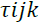Here, the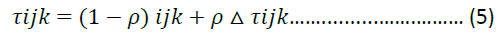is the pheromone value in the point of ?i, j, k?. The ζ is a decay factor.

When the ant completed a path searching, the global function updated the path abases on estimate evaluation. The system chose a shortest path from the sets of path, and adding a number of pheromone into every point of the shortest path. The equal of the pheromone as shown: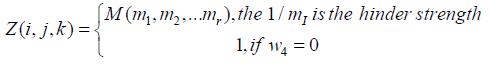Here, the length unit is m. The ρ is the parameter of the pheromone, and the K is the other parameter.

Visual search space: we take the direction of x coordination as the main direction. The agents walk along the direction of x axis. The walking directions of agents have three kinds. The first kind of direction is walking forward; the second kind of direction is walking towards left or right; and the third kind of walking is toward up or down. We supposed that walking forwards is under a unit length distance Ly,max. The maximum lateral movement distance is Ly,max, and the maximum longitudinal movement distance is Lz,max. By this way, when the ant walking along x axis direction, supposed the point is at H?i,j,k?, there is a visual search space.

By this way, when the ant walking from the current point to the next point, the searching area is limited in the visual search area, which simplify the search space and improve the search rate of ant colony algorithm.

Ant colony algorithm search strategy

The next work is making the detail searching strategy and searching process.

1) The improved definition of heuristic function: The heuristic function is the choosing probability of the next step in the visual area (Figure 10).

The heuristic function is:

H (i,j,k) = D (i,j,k)w1* S (i,j,k)w2* Q(i,j,k)w3* Z(i,j,k ) w4..............…..... (6)

The D (i,j,k) is the length of two points, which makes the ant to choice the close points; S(i,j,k) is the safety factor. If the terrain is cliff, the value of S(i,j,k) is 0. This method makes the ant choice the safety points. Q (i,j,k) is rout length, which make the ant choice the closet point near the goal. Z(i,j,k) is the element that is in some place where there is some hinder strength. If there is not any hider strength, then the w4 is 0. Here, w1, w2, w3 is the parameters, which present the important level.

The computational formula of D (i,j,k) is:

D (i,j,k) = sqrt [(xa-xb) 2+(ya-yb) 2+(za-zb)2]…….... (7)

Here, a is the current point, and b is the next point.

The computational formula of S (i,j,k) is:

S(i,j,k)=(Num-Unum)/(Num)…............. (8)

Here, the Num presents the visible point number in the point, and the Unum is the number of the unable to reach area. If the Num-Unum=0, then there are not any passing points.

The computational formula of Q (i,j,k) is?

Q(i,j,k)= sqrt[(xb-xd)2 + (yb-yd)2 + (zb-zd)2]….....…(9)

Here, the b is the next point, and the d is the aim point. The computational formula of Z(i,j,k) is: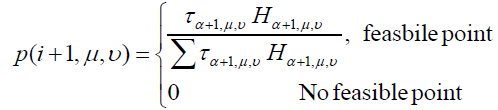................(10)

The classical example is in the military scenario. The best path perhaps is not the best path, because many times there may be some barrier or sniper fire. At this time, the marching path should be as factor in the marching diked areas to plan the marching path. There is the sniper fire area signal as red triangle Area (Figures 11 and 12).

Figure 12: A large-scale mountain environment
The Web3D scene above used the improved 3D ant colony optimal algorithm.

2) Steps for ant colony searching: When an ant in the current pi point of the I plane, the ant would chose the next point in the next plane of II. The steps of the selective probability of pi+1 from the point pi to the point pi+1:

Step 1: Based on the environment visual data to decide the next passing point set in the next plane. The current point is i.

Step 2: Based on the formula 6, calculating the heuristic value H (i+1,u,v) from pi to plane II in turn to find a passing point set.

Step 3: Calculating the p(i+1,u,v) of the any point?i+1,u,v? in the two dimension.in here, the τα+1,μ,υis the pheromone of the point P ?a+1,u,v?in the plane II

Step 4: Based on the selective probability of every point, we use the roulette method to choice the points in the plane II.

The three dimension path planning bases on the ant colony algorithm:

 Initialization algorithm parameters: Give the population size and the best individual Then Initialization pheromone {Calculate the fitness value Get the best fitness value For (passing) Find the best path Update the pheromone } End

Experiment result

In the three dimension route planning, we take use of ant colony algorithm to find the optimal rout. The space model is an area of 20 km × 20 km. we used space planning method to divide the space area into a 20 km × 20 km × 20 km space. Here, along the direction in the X-axis, and the Y-axis, we give every point distance which is 1 km, and the distance between every point is 200 m. The start point corporation of the path is (11,20,810), and the end point corporation is (31,14,1010). The basic design of population size is 20, and the iterate number is 120 times . The results and the best individual fitness satisfaction (Figure 13).

Figure 13: 3D ant colony optimal path planning based on leader idea
The marching soldiers march following the leader. The leader would make path panning based on the real mountain terrain and the enemy fire situation.

As you can see, in the three-dimensional path planning, when the number of iterations is 69, the optimal path is found, at this time, the best individual fitness value is 126 for multi-agent  path finding in large-scale scene. And we can use the ideas of the leader that is using the main agent in the complex path planning , and then sharing the planned path information with other agents, so that the multi-agent following the leader in turn. This kind of using is especially common in the military field. Such as mass marching, the path planning making by commanders, and the other officers and soldiers march according to the commanders who has found the path of the order in a Web3D virtual scene (Figure 14). This kind of method can further save group intelligent path planning resource usage.

Figure 14: Marching soldiers following the leader
All soldiers following the leader (marked in the red circle), and the army walking with a random distance.

## Conclusion

This paper has put forward multi-agent ant colony optimal in 2D and 3D path planning scheme. It has the following advantages: 1) It has put forward a complete 2D and 3D ant colony path planning scheme; 2) It has made a 2D ant colony search of point selection of optimization on the road. This can accelerate the speed of the optimal path finding, and can make use of the structure of the edge of linear features to find the shortest path. 3) It has proposed a vertebral viewing area planning in 3D ant colony path finding calculation and has provided an effective method for the agent to select the next step node. This accelerates the process of path finding. In the heuristic function structure, considering the actual situation often has hinder area, we set the resistance element to guarantee practical applications; 4) In view of the practical situation of 2D or 3D terrain scene, we put forward the starting point and end point of attachment on the map in the direction map according to the global path planning to identify 2D or 3D terrain path finding method; 5) On the basis of calculating the premise of limited resources, we proposed the leader idea thought the ant colony in 2D or 3D path optimization calculation. When we calculate the path of a leader agent, other agents would follow the leader. By this way, it can improve the computational efficiency in the large-scale scene for multi-agent path planning to reduce the computing resources usage. The existing shortcomings are as follows: 1) in 2D path planning, if emergencies occur, such as fire, agents within the scene will not be able to accurately judge the coverage with fire. It only can determine a roughly range, probably just an escaping path planning; 2) in 3D path planning, if the scene is too big, there will be more iterations to find the optimal path.

The next step of work, we will put 2D and 3D path planning algorithm into application of the path planning in web virtual scene, and the path planning in mobile devices.

## Acknowledgements

This work is partially supported by Jing gang Mountain National Fund Project 2012BAC11B01-04.

Get the App